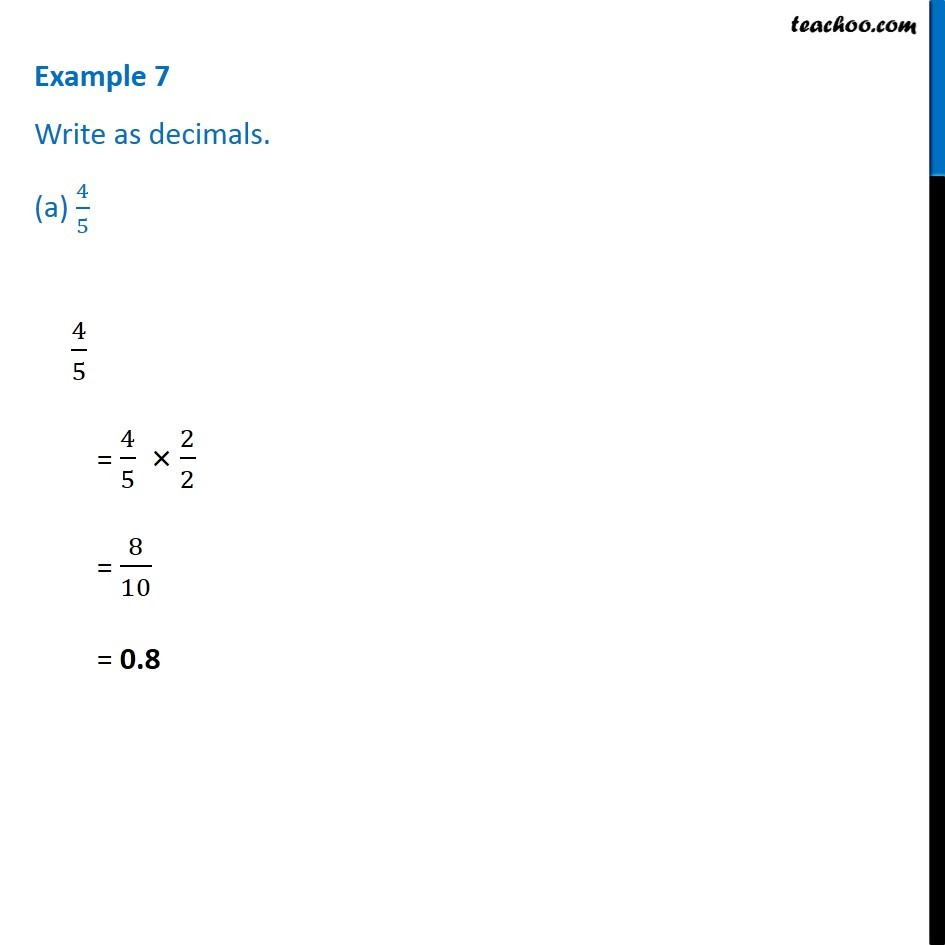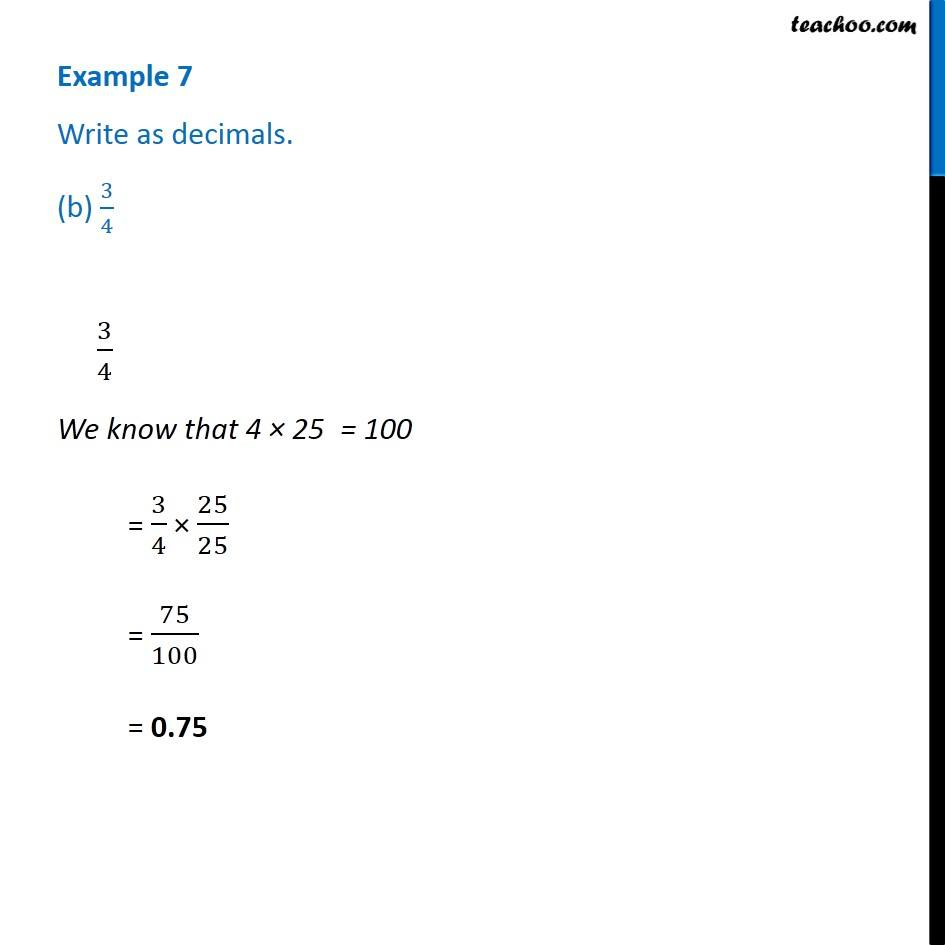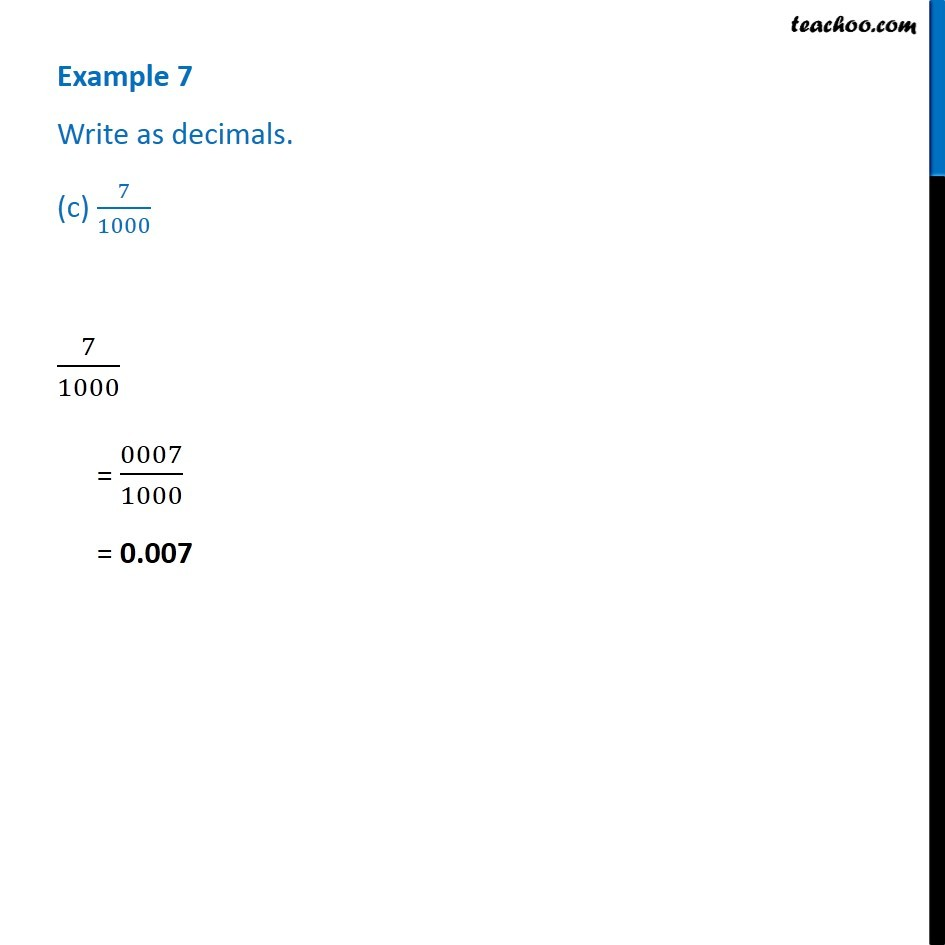Subscribe to our Youtube Channel - https://you.tube/teachoo

1. Chapter 8 Class 6 Decimals
2. Serial order wise
3. Examples

Transcript

Example 7 Write as decimals. (a) 4/5 4/5 = 4/5 2/2 = 8/10 = 0.8 3/4 We know that 4 25 = 100 = 3/4 25/25 = 75/100 = 0.75 Example 7 Write as decimals. (c) 7/1000 7/1000 = 0007/1000 = 0.007

Examples

Chapter 8 Class 6 Decimals
Serial order wise